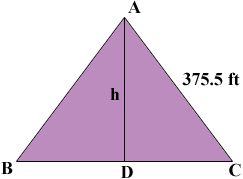SEARCH HOMEMath Central Quandaries & QueriesQuestion from kylie, a student: the vertex angle of an isosceles triangle is 57 degrees 24 minutes and each of its equal sides is 375.5 feet long. find the altitude of the triangleHi Kylie,In my diagram AD is perpendicular to BC so the length of AD is the altitude of the triangle ABC. Since the triangle is isosceles angles ABC and BCA are congruent. The angles CAD and DAB are also congruent. Do you see why? What is the measure of angle DAB? Using the triangle ADC what trig function relates the measure of the angle CAD, the length of AD and the length of CA?

I hope this helps,
PennyMath Central is supported by the University of Regina and The Pacific Institute for the Mathematical Sciences.